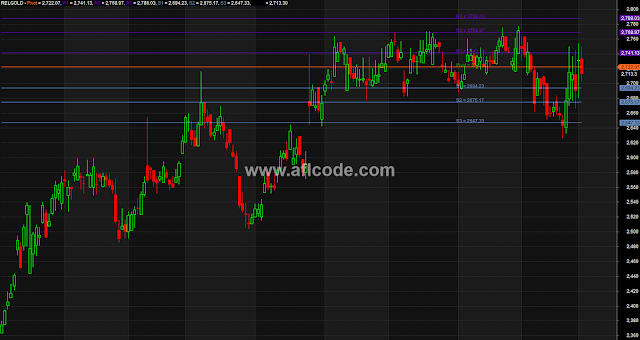### Three Level Continuous Pivot PointsThree Level Continuous Pivot Points
```//www.aflcode.com
h1= SelectedValue (Ref(H,0) );
l1= SelectedValue (Ref(L,0) );
c1= SelectedValue (Ref(C,0) );

// PIVOT Calculation
p = ( H1+ L1 + C1 )/3;
s1 = (2*p)-H1;
r1 = (2*p)-L1;
s2 = p -(H1 - L1);
s3 = S1 - (H1-L1);
r2 = p +(H1 - L1);
r3 = R1 +(H1-L1);

// PIVOT mid points
MIDR1 = (P+R1)/2;
MIDR2 = (R1+R2)/2;
MIDR3 = (R2+R3)/2;
MIDS1 = (P+S1)/2;
MIDS2 = (S1+S2)/2;
MIDS3 = (S2+S3)/2;

// Graphs & values plot
Plot (p,"Pivot",25,1);
Plot (r1,"R1",12,1);
Plot (r2,"R2",12,1);
Plot (r3,"R3",12,1);
Plot (s1,"S1",3,1);
Plot (s2,"S2",3,1);
Plot (s3,"S3",3,1);

/*
Plot (MIDR1,"midr1",12,32);
Plot (MIDR2,"midr2",12,32);
Plot (MIDR3,"midr3",12,32);
Plot (MIDS1,"mids1",12,32);
Plot (MIDS2,"mids2",12,32);
Plot (MIDS3,"mids3",12,32);
*/

Plot (C,"Close",16,64);
// side might be ajusted depends on data array
Hight =33;
side = Param("side",73,0,1000,1);

for( i = 0; i < BarCount; i++ )
{
if(i+side== BarCount) PlotText( "Pivot = "+P, i+Hight,P, colorGreen );
if(i+side== BarCount) PlotText( "R1 = "+R1, i+Hight,R1, 12 );
if(i+side== BarCount) PlotText( "R2 = "+R2, i+Hight,R2, 12 );
if(i+side== BarCount) PlotText( "R3 = "+R3, i+Hight,R3, 12 );
if(i+side== BarCount) PlotText( "S1 = "+S1, i+Hight,S1, 3);
if(i+side== BarCount) PlotText( "S2 = "+S2, i+Hight,S2, 3 );
if(i+side== BarCount) PlotText( "S3 = "+S3, i+Hight,S3, 3 );
}

// text section

"HIGH /LOW /CLOSE = " +H +" / "+ L+" / "+ C +"\n";
"H1 /L1 /C1 = " +H1 +" / "+ L1+" / "+ C1 +"\n";

"R3 = " +R3;
"midr3 = " +MIDR3;
"R2 = " +R2;
"midr2 = " +MIDR2;
"R1 = " +R1;
"midr1 = " +MIDR1;
"*************";
"p = " +p;
"*************";
"mids1 = " +MIDS1;
"S1 = " +S1;
"mids2 = " +MIDS2;
"S2 = " +S2;
"mids3 = " +MIDS3;
"S3 = " +S3;
```
Previous
Next Post »